• 1 数组的概念 数组可以看成是多相同类型数据组合，这些数据的统一管理。 数组变量属引用类型，数组也可以看成是对象，...一维数组的声明方式：type var[]或type[] var 　例如：int a1[]; int[] a2; 　double　b[]
1 数组的概念

数组可以看成是多个相同类型数据组合，对这些数据的统一管理。
数组变量属引用类型，数组也可以看成是对象，数组中的每个元素相当于该对象的成员变量。
数组中的元素可以是任何数据类型，包括基本类型和引用类型。
2 一维数组的声明

一维数组的声明方式：type var[]或type[] var
例如：int a1[];   　　int[] a2;
double　b[];
Person[] p1; String s1[];
Java语言中声明数组时不能指定其长度（数组中元素的个数），例如：int a;//非法
Java中使用关键字new 创建数组对象，格式为：数组名 = new 数组元素的类型[数组元素的个数]
    public class Test {
public static void main(String[] args){
int[] s;
s = new int;
for (int i=0; i < 5;i++){
s[i] = i;
}
}
}

元素为引用数据类型的数组中的每一个元素都需要实例化。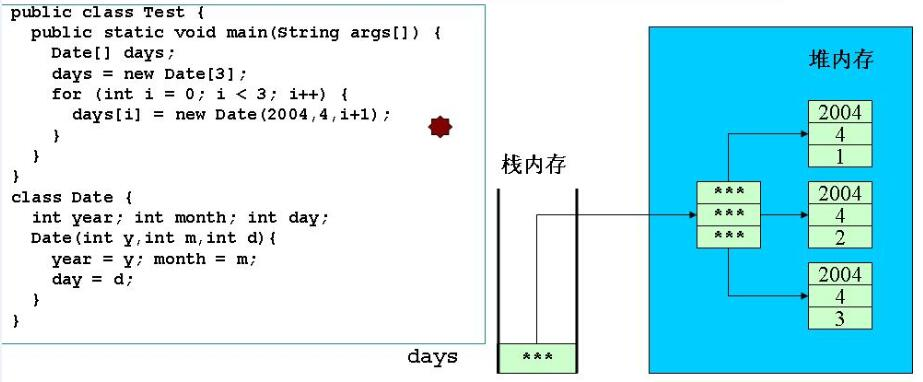3 一维数组的初始化

动态初始化：数组定义与为数组元素分配空间和赋值的操作分开进行，先分配空间再赋值。
    public class Test {
public static void main(String[] args){
int[] a;
a = new int;
for (int i=0;i<3;i++){
a[i]=i;
}
Date[] day;
day = new Date;
for (int i=0;i<3;i++){
day[i]= new Date(2016,10,i+1);
}
}
}

class Date{
int year;int month;int day;
Date(int y,int m,int d){
year = y; month = m; day = d;
}
}

静态初始化：在定义数组的同时就为数组元素分配空间并赋值。
    public class Test {
public static void main(String[] args){
int[] a = {3,9,8};
Date[] day = {new Date(2016,10,1),
new Date(2016,10,2),new Date(2016,10,3)};
}
}
}

class Date{
int year;int month;int day;
Date(int y,int m,int d){
year = y; month = m; day = d;
}
}

4 数组元素的默认初始化
数组是引用类型，它的元素相当于类的成员变量，因此数组分配空间后，每个元素也被按照成员变量的规则被隐式初始化。

    public class Test {
public static void main(String[] args){
int[] a = new int;
Date[] days = new Date;
System.out.println(a);//0
System.out.println(days);//null
}
}

class Date{
int year;int month;int day;
Date(int y,int m,int d){
year = y; month = m; day = d;
}
}


5 数组元素的引用

定义并用运算符new为之分配空间后，才可以引用数组中的每个元素。
每个数组都有一个**属性**length指明它的长度，例如a.length的值为数组a的长度（元素个数），不是方法，与String的length()做区别。
选择排序（从小到大）：遍历一次找出最小的数，放到起始位置

int类型排序：

public class NumSort{
public static void main(String[] args){
int[] a = new int[args.length];
for (int i=0;i<args.length;i++){
a[i]=Integer.parseInt(args[i]);
}
print(a);
//selectSort(a);
bubbleSort(a);
print(a);
}
//选择排序
private static void selectSort(int[] a){
int k,temp;
for(int i=0;i<a.length;i++){
k=i;
for(int j=k+1;j<a.length;j++){
if (a[k]>a[j]){
k = j;
}
}
if (k!=i){
temp = a[i];
a[i] = a[k];
a[k] = temp;
}
}
}
//冒泡排序
private static void bubbleSort(int[] a){
int len=a.length;
for(int i=len-1;i>=1;i--){
for (int j=0;j<=i-1;j++){
if (a[j]>a[j+1]){
int temp=a[j];
a[j]=a[j+1];
a[j+1]=temp;
}
}
}
}

private static void print(int[] a){
for(int i=0;i<a.length;i++){
System.out.print(a[i]+" ");
}
System.out.println();
}
}

运行结果：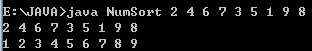引用对象的排序：

public class TestDataSort{
public static void main(String[] args){
Date[] days = new Date;
days = new Date(2006,5,4);
days = new Date(2006,7,4);
days = new Date(2008,5,4);
days = new Date(2004,5,9);
days = new Date(2004,5,4);

bubbleSort(days);
//selectSort(days);

for(int i=0;i<days.length;i++){
//直接打印对象的引用会调用toString
System.out.println(days[i]);
}
}
//冒泡排序
public static Date[] bubbleSort(Date[] a){
int len = a.length;
for (int i=len-1;i>=1;i--){
for(int j=0;j<=i-1;j++){
//如果a[j]>a[j+1]
if (a[j].compare(a[j+1])>0){
Date temp = a[j];
a[j]=a[j+1];
a[j+1]=temp;
}
}
}
return a;
}
//选择排序
public static Date[] selectSort(Date[] a){
int k;
Date temp;
for (int i=0;i<a.length;i++){
k=i;
for (int j=k+1;j<a.length;j++){
if (a[k].compare(a[j])>0){
k=j;
}
}
if (k!=i){
temp=a[i];
a[i]=a[k];
a[k]=temp;
}
}
return a;
}
}

class Date{
int year,month,day;
Date(int y,int m,int d){
year=y;
month=m;
day=d;
}
public int compare(Date date){
return year > date.year ? 1
: year < date.year ? -1
: month > date.month ? 1
: month < date.month ? -1
: day > date.day ? 1
: day < date.day ? -1:0;
}
//重写toString()
public String toString(){
return"Year:Month:Day --"+year+"-"+month+"-"+day;
}
}

运行结果：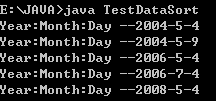二分法： 找到12所在的位置

public class TestSearch{
public static void main(String[] args){
//二分法一定要在排好序的前提下进行
int a[] = {1,3,6,8,9,10,12,18,20,34};
int i = 12;
System.out.println(binarySearch(a,i));
}

public static int search(int[] a,int num){
for (int i=0;i<a.length;i++){
if(a[i] == num)
return i;
}
return -1;
}

public static int binarySearch(int[] a, int num){
if (a.length == 0){
return -1;
}
int startPos =0;
int endPos = a.length-1;
int m=(startPos+endPos)/2;
while (startPos<= endPos){
if (num ==a[m]){
return m;
}
if (num > a[m]){
startPos = m+1;
}
if (num < a[m]){
endPos = m-1;
}
m = (startPos + endPos)/2;
}

return-1;
}

}

6 二维数组
二维数组可以看成以数组为元素的数组，例如：int [][] ={{1,2}，{3,4,5,6}，{7,8,9}};
Java中多维数组的声明和初始化应按从高维到低维（从左到右）的顺序进行，例如：

    int a[][] = new int[];
a = new int;
a = new int;
a = new int;
int t1[][] = new int[];//非法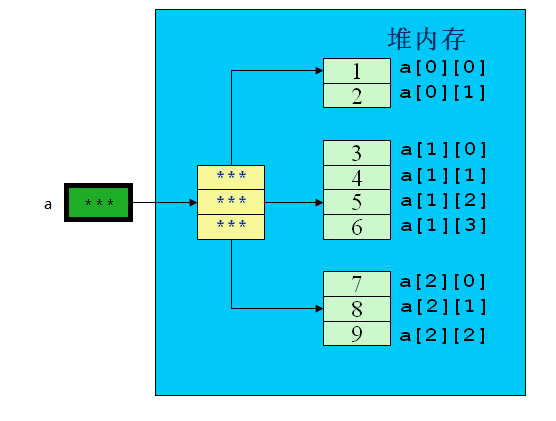静态初始化：int A[][] = {{1,2},{2,3},{3,4,5}};

动态初始化：

    int a[][] = new int;
int b[][] = new int[];
b = new int;
b = new int;
b = new int;

例子程序：

public class TestArray{
public static void main(String[] args){
int a[][]={{1,2},{3,4,5,6},{7,8,9}};
for (int i=0;i<a.length;i++){
for(int j=0;j<a[i].length;j++){
System.out.print("a["+i+"]["+j+"]=" +a[i][j]+" ");
}
System.out.println();
}
}
}

运行结果：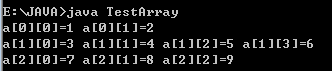String类型的二维数组：

public class TestString{
public static void main(String[] args){
String[][] s = new String[];
s = new String;
s = new String;
s = new String;
for (int i =0;i<s.length;i++){
for(int j=0;j<s[i].length;j++){
s[i][j] =new String("我的位置是："+i+","+j);
}
}
for(int i=0;i<s.length;i++){
for (int j=0;j<s[i].length;j++){
System.out.print(s[i][j]+" ");
}
System.out.println();
}
}
}

运行结果：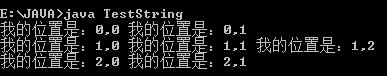数组的拷贝：

使用java.lang.System类的静态方法
public static void arraycopy(Object src,int srcPos,Object dest,int destPos,int length);

可以用于数组src从第srcPos项元素开始的length个元素拷贝到目标数组从destPos项开始的length个位置
如果源数据数目超过目标数组边界会抛出IndexOutOfBoundsException异常。
例子程序：

public class TestArrayCopy{
public static void main(String[] args){
String[] s ={"Microsoft","IBM","Sun","Oracle","Apple"};
String[] sBak = new String;
System.arraycopy(s,0,sBak,0,s.length);

for (int i=0;i<sBak.length;i++){
System.out.print(sBak[i]+" ");
}

System.out.println();
int[][] intArray = {{1,2},{1,2,3},{3,4}};
int[][] intArrayBak = new int[];
System.arraycopy(intArray,0,intArrayBak,0,intArray.length);
intArrayBak = 100;
//虽然没有直接对intArray进行操作，但intArray的值也会发生变化
for(int i=0;i<intArray.length;i++){
for(int j=0;j<intArray[i].length;j++){
System.out.print(intArray[i][j]+" ");
}
System.out.println();
}
}
}

运行结果：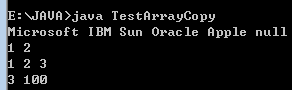展开全文• 、基本概念 （1）数组是多相同类型数据的组合，实现这些数据的统一管理 ...一维数组的相关介绍 通过代码的形式来介绍一维数组的声明、初始化等相关注意 public class ArrayTest { public sta...
一、基本概念

（1）数组是多个相同类型数据的组合，实现对这些数据的统一管理

（2）数组中的元素可以是任何数据类型，包括基本类型和引用类型

（3）数组属引用类型，数组型数据是对象(object)，数组中的每个元素相当于该对象的成员变量

二、一维数组的相关介绍

通过代码的形式来介绍一维数组的声明、初始化等相关注意

public class ArrayTest {
public static void main(String[] args) {
// 1.数组的声明和初始化
int[] arr1; // 数组的声明
// 静态初始化：数组的初始化和数组元素的赋值同时进行
arr1 = new int[] { 1, 2, 3, 4 };
// 合并声明和初始化
int[] arr2 = new int[] { 4, 5, 6, 7 };
//或：int arr2[] = new int[] { 4, 5, 6, 7 };
//或：int[] arr2 = {4, 5, 6, 7}; //类型推断

// 动态初始化：数组的初始化和数组元素的赋值分开进行
String[] arr3 = new String;

//2.如何调用数组的元素：通过角标的方式。角标从0开始，到长度-1结束
arr3="文静";
arr3="文明";
arr3="文化";

//错误的声明方式
//int[] arr4 = new int {1,2,3,4};
//int arr4 = new int[]{1,2,3,4};

//3.数组的长度:使用length属性调用
System.out.println(arr2.length);//4
System.out.println(arr3.length);//4

//4、遍历数组的元素
for(int i = 0;i<arr3.length;i++) {
System.out.println(arr3[i]);
}
//5.1整型数组元素的默认初始化值为：0
//5.2浮点型数组元素的默认初始化值为：0.0
//5.3字符型数组元素的默认初始化值为：‘\u0000’或0，不等同于'0'
//5.4布尔类型数组元素的默认初始化值为：false
//5.5引用数据类型数组元素的默认初始化值为：null,不等于“null”
}

}

二、二维数组的相关介绍

public class MoreArrayTest {
public static void main(String[] args) {
//		1、二维数组的声明和初始化
// 静态初始化：数组的初始化和数组元素的赋值同时进行
int[][] arr1 = new int[][] { { 1, 2, 3 }, { 4, 5 }, { 7, 8 } };

// 动态初始化：数组的初始化和数组元素的赋值分开进行
// 动态初始化方式1：
int[][] arr2 = new int;
// 动态初始化方式2：
int[][] arr3 = new int[];
arr3 = new int;
arr3 = new int;
arr3 = new int;

// 错误的初始化方式
//		int[][] arr4 = new int[];
//		int[][] arr5 = new int {{1,2},{3,4},{5,6}};

//		2.调用数组元素使用角标的形式
System.out.println(arr1);
//		3.数组的长度
System.out.println(arr1.length);// 3
System.out.println(arr1.length);// 2
//		4.二位数组的遍历
for (int i = 0; i < arr1.length; i++) {
for (int j = 0; j < arr1[i].length; j++) {
System.out.println(arr1[i][j]);
}
}
}
}

三、杨辉三角问题

public class YangHuiTest {
public static void main(String[] args) {
// 创建数组
int[][] yangHui = new int[];

for (int i = 0; i < yangHui.length; i++) {
yangHui[i] = new int[i + 1];
// 首末位置赋值为一
yangHui[i] = yangHui[i][i] = 1;
//第三行开始，除了首末位置为1外，其余位置的数字，是所这个数字对应（j列）的上一行（i-
//1行）的数字，与上一行（i-1行）对应的数字前一个数字(j-1列)之和
for (int j = 1; j < yangHui[i].length - 1; j++) {
yangHui[i][j] = yangHui[i - 1][j] + yangHui[i - 1][j - 1];
}
}
//遍历数组
for(int i =0;i<yangHui.length;i++) {
for(int j=0;j<yangHui[i].length;j++) {
System.out.print(yangHui[i][j]+"\t");
}
System.out.println();
}
}
}


输出结果：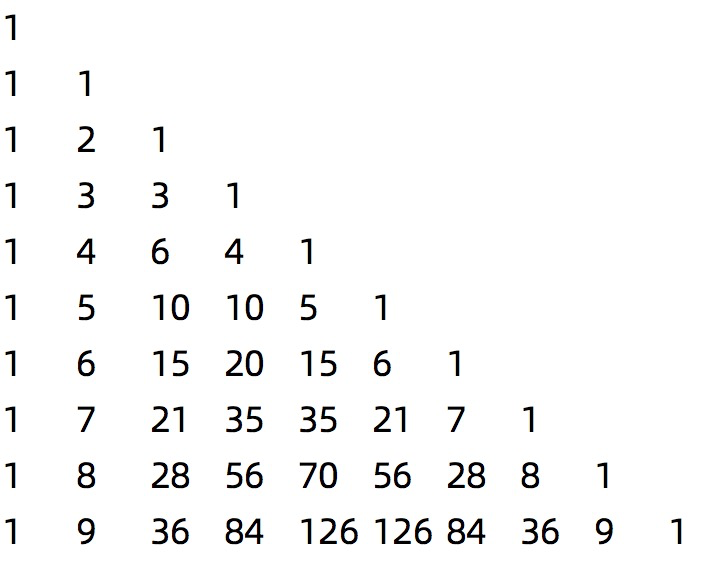展开全文java
• 数组的元素可以是任何数据类型，包括基本数据类型和引用数据类型。 数组属引用类型，数组型数据是对象，数组中的每元素相当于该对象的成员变量。 数组是多相同类型数据的组合，实现这些数据的统一管理。 ...
介绍数组

Java 中的数组是用来存储固定大小的同类型元素。
数组中的元素可以是任何数据类型，包括基本数据类型和引用数据类型。
数组属引用类型，数组型数据是对象，数组中的每个元素相当于该对象的成员变量。
数组是多个相同类型数据的组合，实现对这些数据的统一管理。
数组主要解决多变量多数据存储的问题，方便程序后期统一维护操作数据。
数组就是一系列空间大小相等且地址连续的一片存储空间。
数组变量存的就是数组在堆内存中首元素的地址。
数组存在于堆内存中，但凡在堆内存中存储的数据都称之为对象，但凡在堆内存中创建的对象都会有默认初始值，整数类型默认0，浮点类型默认0.0，布尔类型默认false,引用数据类型（对象）默认null.

声明数组

元素类型[] 数组名; // 建议使用的方式

元素类型 数组名[];  

int[] score = null;  // null表示引用数据类型的默认值
int socre[] = null;  

注意：

首先必须声明数组变量，才能在程序中使用数组。
“数组名”是用来统一这组相同数据类型的元素的名称，建议使用有意义的名称为数组命名。

开辟空间

数组名 = new 元素类型[元素的个数];
score = new int;

如图所示：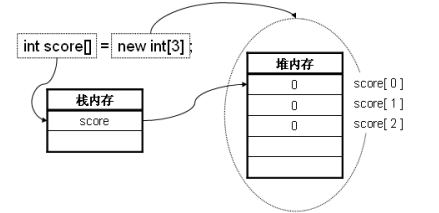注意：

数组操作中，在栈内存中保存的永远是数组的名称，只开辟了栈内存空间数组是永远无法使用的，必须有指向的堆内存才可以使用，要想开辟新的堆内存则必须使用new关键字，之后只是将此堆内存的使用权交给了对应的栈内存空间，而且一个堆内存空间可以同时被多个栈内存空间指向，即：一个人可以有多个名字，人就相当于堆内存，名字就相当于栈内存。

数组的初始化(创建数组时必须明确规定大小或内容）

元素类型 数组名 = new 元素类型[数组大小];
元素类型 数组名[] = new 元素类型[]{1,2,3,4};
元素类型 [] 数组名={值0，值1，值2....}
int[] score = new int;
double[]myList=new double;
double []myList={1.9,2.9,3.4,3.5};

在Java中，由于整数数据类型所占用的空间为4个bytes，而整型数组score可保存的元素有10个，所以上例中占用的内存共有4 * 10 = 40个字节。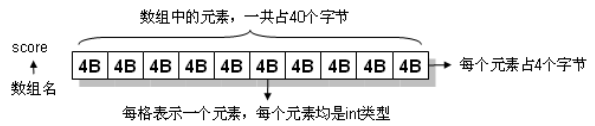public class ArrayTest {
public static void main(String[] args) {
// 对各元素进行初始化，但没有赋值。
String[] stringArray = new String; // 各元素的值默认为String类型的初始值null
// 数组没有length()这个方法，有length的属性。
for (int i = 0; i < stringArray.length; i++) {
System.out.println(stringArray[i]);  // null null null
}
}
}
数组是引用类型，它的元素相当于类的成员变量，因此数组一经分配空间，其中的每个元素也被按照成员变量同样的方式被隐式初始化。

数组中元素的表示方法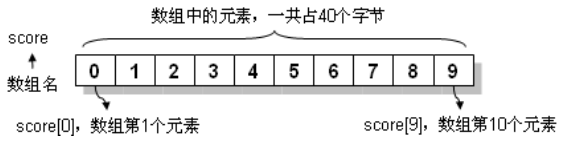Java的数组索引编号由0开始，以一个的score的整形数组为例，score代表第1个元素，score代表第2个元素，score为数组中第10个元素（也就是最后一个元素）.数组通过角标来访问元素的具体计算方式是 所要访问数据的地址=首元素地址+角标*数据类型大小

注意：数组下标越界的错误提示：java.lang.ArrayIndexOutOfBoundsException。NullPointerException 空指针异常.

数组的基本操作

1.使用随机数初始化数组

for(int i=0;i<myList.length;i++){
myList[i]=Math.random()*100;
}

2.显示数组

for(int i=0;i<myList.length;i++){
System.out.print(myList[i]+" ");
}

3.对所有元素求和

double total=0；
for（int i=0；i<=myList.length;i++){
total+=myList[i];
}

4.找出最大元素

double max =myList;
for(int i=1;i<myList.length;i++){
if(myList[i]>max){
max=myList[i];
}
}

5.找出最大元素的最小下标值

double max =myList;
int indexOfMax=0;
for(int i=1;i<myList.length;i++){
if(myList[i]>max){
max=myList[i];
indexOfMax=i;
}
}

数组的查找操作（线性查找，二分查找）

1.二分查找

public static void binarySearch(){
/*
最好情况 查46 1次就出来了
最坏情况 查12/60 O(logn)
*/
int[] arr={12,17,21,32,38,41,46,49,50,50,51,59,60};
int key=46;
int index=-1;
int min_index=0;
int max_index=arr.length-1;
int mid_index=(min_index+max_index)/2;
while(arr[mid_index]!=key){
if(key<arr[mid_index]){
max_index=mid_index-1;
}
if(arr[mid_index]<key){
min_index=mid_index+1;
}
if(min_index>max_index){
index=-1;
break;
}
mid_index=(min_index+max_index)/2;
}
System.out.println(mid_index);

}

注意：二分查找有个前提 数组必须有序。

2.线性查找

public static void linearSearch(){
/*
最好情况 查10 1次就出来了
最坏情况 查5  10次才出来
当数组的长度越大的话 最坏情况越差
时间复杂度(最坏情况) O(n) 线性阶
*/
int[] arr={10,2,8,3,1,6,4,7,9,5};
int key=11;
int index=-1;   //key元素不存在
for(int i=0;i<arr.length;i++){
if(arr[i]==key){
index=i;
break;
}
}
System.out.println(index);
}

二维数组

就是一个由行和列组成的一个表格而已，矩阵Matrix.
在矩阵中访问元素的的话，是根据元素的行角标和列角标所确定的。
尤其对于二维数组而言，无非就是一个一维数组，只不过该一维数组中的每一个元素是另一个一维数组罢了！

二维数组中数据的存储：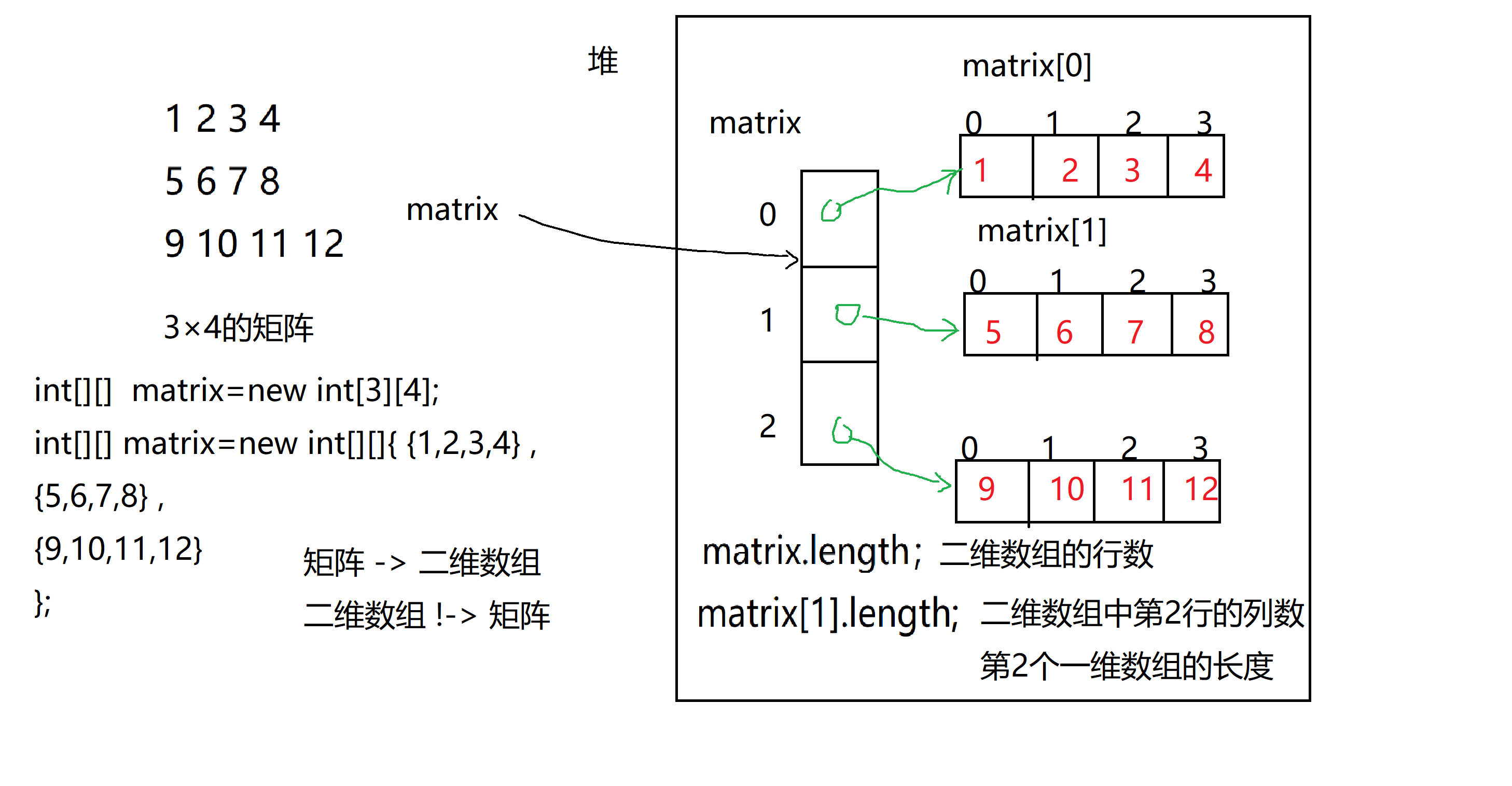这个图中共有四个数组，分别是matrix, matrix, matrix, matrix。 matrix.length;表示二维数组的行数为三，matrix.length;表示二维数组中第二行数组的长度。

二维数组的创建

1.   int[][]  matrix=new int;---------------创建一个3行4列的二维数组，元素默认都是0

2.   int[][] matrix=new int[][]{ ---------------创建个指定元素的二维数组

{1,2,3,4} ,

{5,6,7,8} ,

{9,10,11,12}

};

3.   int[][] matrix={

{1,2,3,4} ,

{5,6,7,8} ,

{9,10,11,12}

};

4.   锯齿矩阵/二维数组

int[][] matrix={

{1,2,3,4} ,

{5,6,7} ,

{8,9},

{10}

};

展开全文java c语言
• 在php中数组排序一直是一个老生常谈问题，下面我们来集中讲一下关于在php中一维数组与二维数组排序实现程序，相信大家有一定参考借鉴价值. 功能:对数组进行重新排序. 说明:冒泡排序 (一维数组)(二维数组某个...
• 数组：是一个存储相同类型元素且固定大小顺序集合。 数组声明： datatype[] arrayName; 数组类型是从抽象基类型 Array 派生引用类型。由于此类型实现了 IEnumerable ，因此可以 C# 中所有数组使用 ...
数组：是一个存储相同类型元素且固定大小的顺序集合。
数组声明：
datatype[] arrayName;
数组类型是从抽象基类型
Array 派生的引用类型。由于此类型实现了 IEnumerable ，因此可以对 C# 中的所有数组使用 foreach 迭代,foreach循环对数组内容进行只读访问，所以不能改变任何元素的值。

//一维数组
static void Main(string[] args)
{
int[] a = { 1,2,3,3}; //初始化
int[] b = new int[] { 1,2,3,4,5};//初始化
//var c= a.Intersect(b);  //求交集
var c = b.Except(a); //求差集
foreach (var item in c)
{
Console.WriteLine(item);
}
Console.WriteLine("数组A的平均值："+ a.Average());
Console.Read();
}
//二维数组 同 多维数组 又称矩形数组
static void Main(string[] args)
{
int[,] myArray;
myArray = new int[2, 3];//2行 3列
myArray[0, 0] = 1;
myArray[0, 1] = 2;
myArray[0, 2] = 3;
myArray[1, 0] = 4;
myArray[1, 1] = 5;
myArray[1, 2] = 6;
foreach (var item in myArray)
{
Console.WriteLine(item);
}
Console.ReadLine();
}
//锯齿数组 又称交错数组
static void Main(string[] args)
{
//声明一个锯齿数组  三行
int[][] myArray;
myArray = new int[];
myArray = new int[] { 1,2,3,4,5};
myArray = new int { 1,4,6};
myArray = new int[] { 2,3,4,5};
//Console.WriteLine(myArray); 第二行，第四个数，不存在，会报错：超出索引
//数据输出如下：
for (int i = 0; i < myArray.Length; i++)
{
Console.WriteLine("第"+(i+1)+"行");
for (int j = 0; j < myArray[i].Length; j++)
{
Console.Write(myArray[i][j]+",");
}
Console.WriteLine();

}
Console.ReadLine();
}


展开全文c#
• 对一个没有确定row的二维数组，怎么将其作为参数呢？一维数组，可以直接用指针作为参数，而二维数组却并不是指针的指针，任何多维数组和任何形式的指针无法简单转换（隐式或显式）。我的一个经验是取静态多维数组...
• * 数组一个源自底层数据结构,并且几乎在任何语言中,都是最基础数据结构 数组 又称为 索引数组(index) 数据结构 * 数据结构 : * 就是计算机数据存储形式,和数据操作形式 * 精心选择数据结构...java
• 、数组概述  1. 数组可以看成是多相同类型数据组合，这些数据的统一管理。  2. 数组变量属引用类型，数组也可以看成是...一维数组的声明  1.一维数组的声明方式： type var[ ] ; 或 type[ ] var ;  2.java
• 今天我们要开始来讲讲Java中的数组,包括维数组和二维数组的静态初始化和动态初始化 数组概述: 数组可以看成是多相同类型数据的组合,这些数据的统一管理; 数组变量属于引用数据类型,数组也可以看成是对象,...
• 维数组的定义 具有一个下标的数组称为一维数组 定义一维数组的形式如下： 数据类型 数组名 [常量表达式] 例如： int a; 注意： 数据类型指数组的数据类型，也就是每一个数组元素的数据类型。可以是任何合法的...
• 它是方阵，从左上角到右下角的角线（称为主角线）上的元素均为1。除此以外全都为0。根据单位矩阵的特点，任何矩阵与单位矩阵相乘都等于本身 输出a单位矩阵 #include &lt;std...对称矩阵
• 冒泡排序及二维数组 一.冒泡排序 算法步骤：比较相邻的元素。如果第一个比第二个大，就交换他们两个。 每一对相邻元素作同样的工作，从开始第一到结尾的最后一对。这步做完后，最后的元素会是最大的数。 针对...
• /** * @param args * @author wangxianpeng * * 工作原理： 比较相邻的元素， 如果第一个比第个大， 就交换它们两个。 * 每一对相邻元素做同样的工作，从开始第一个对到结尾最后一对。最后 * 的元素应该会是最大...
• 使用Java中维数组模拟战舰游戏这是一个课堂项目，旨在扩大我们数据结构理解 使用算法进行数组分配。 使用3维数组创建3D战舰程序。 将有5艘船将隐藏在每个关卡的任何位置。 该板将是3 X 10 X 10 为每艘船...Java
• ## JavaSE数组

千次阅读 多人点赞 2020-11-27 20:46:14
JavaSE数组维数组数组的定义数组的创建数组的初始化数组赋值数组的遍历数组的异常二维数组数组的声明、创建、初始化二维数组的遍历 数组可以看成是多相同类型数据的组合，实现这些数据的统一管理。 维数组...java
• ## 数组

2020-12-17 13:37:01
数组的元素可以是任何数据类型，包括基本类型和引用类型。 C和C++中的数组都可以分配在栈上面，而JAVA中的数组是只能分配在堆上面的，因为JAVA中的数组是引用类型。 维数组 一维数组的声明方式有2种： ...
• 对任何一个二维数组的元素，都可以用数组名唯一地加以确定。对还是错。求解释。c
• 任务编写冒泡排序 冒泡排序算法的原理如下： 1.比较相邻的元素。如果第一个比第个大，就交换他们两个。 2.每一对相邻元素做同样的...4.持续每次越来越少的元素重复上面的步骤，直到没有任何一对数字需要比较。java
• 数组(Array)，是多个相同类型数据按一定顺序排列的集合，并使用一个名字命名，并通过编号的方式这些数据进行统一管理。 数组的常见概念 数组名 下标(或索引) 元素 数组的长度 数组本身是引用数据类型，而数组...java
• 不带任何下标的二维数组名表示二维数组的起始地址. 只带一维下标的二维数组名表示作为其元素一个一维数组（即二维数组一行）的起始地址，其进行加法操作时表示该一维数组（即二维数组一行）的一个元素的地址。 ...
• 1.数组的概述    数组英文：array。数组是多相同类型数据的组合，实现这些数据的统一管理 数组中的元素可以是任何数据类型，包括基本类型和引用类型。数组属引用类型，数组型数据是对象(object)...二维数组...
• java中的数组一、数组的概述二、一维数组三、二维数组四、Arrays工具类的使用五、数组中常见的异常 一、数组的概述 1、数组(Array)，是多个相同类型数据按一定顺序排列的集合，并使用一个名字命名，并通过编号的方式...java 数据结构 编程语言...